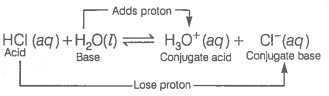The ionisation of hydrochloric acid in water is given below

$\mathrm{HCl}\left(\mathrm{aq}\right)+{\mathrm{H}}_{2}\mathrm{O}\left(\mathrm{l}\right)⇌{\mathrm{H}}_{3}{\mathrm{O}}^{+}\left(\mathrm{aq}\right)+{\mathrm{Cl}}^{-}\left(\mathrm{aq}\right)$

Label two conjugate acid-base pairs in this ionisation.